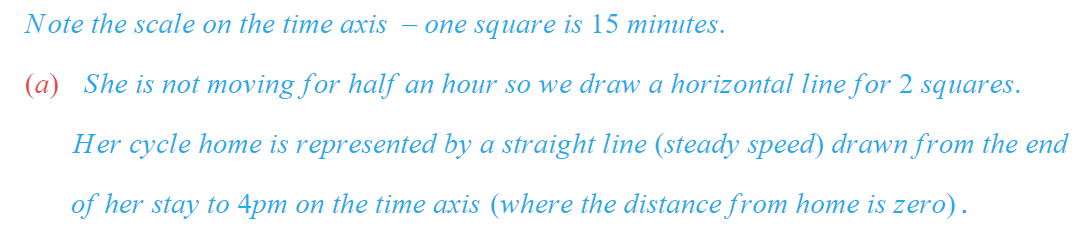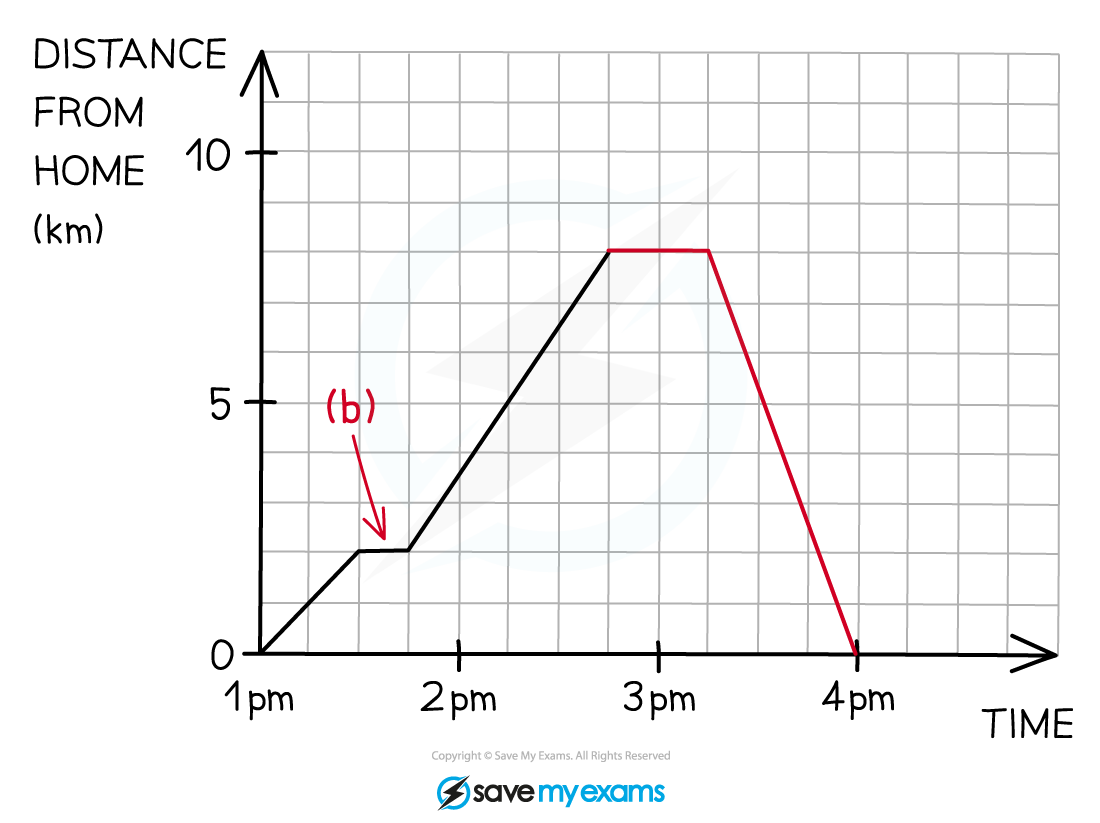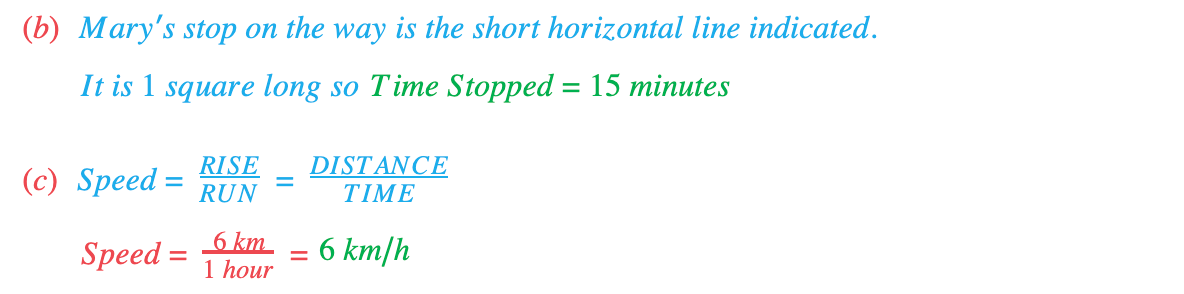# Edexcel IGCSE Maths 复习笔记 3.3.1 Distance-Time Graphs

#### What is a distance-time graph?

• Distance-time graphs show distance from a fixed point at different times
• Distance is on the vertical axis, and time is on the horizontal axis.

• The gradient of the graph is the speed:Speed =RISE / RUN=DISTANCE / TIME
• Straight line = Constant speed
• Horizontal line = Stationary (not moving!)

#### Exam Tip

It is easy to get confused between different types of graph.

Look at the label on the vertical axis to make sure you are looking at a DISTANCE-time graph (not speed-time).

#### Worked Example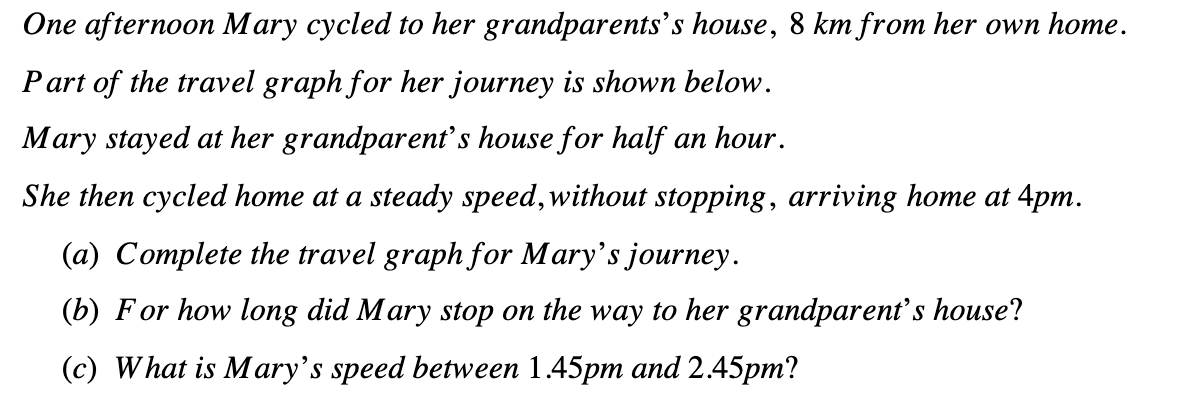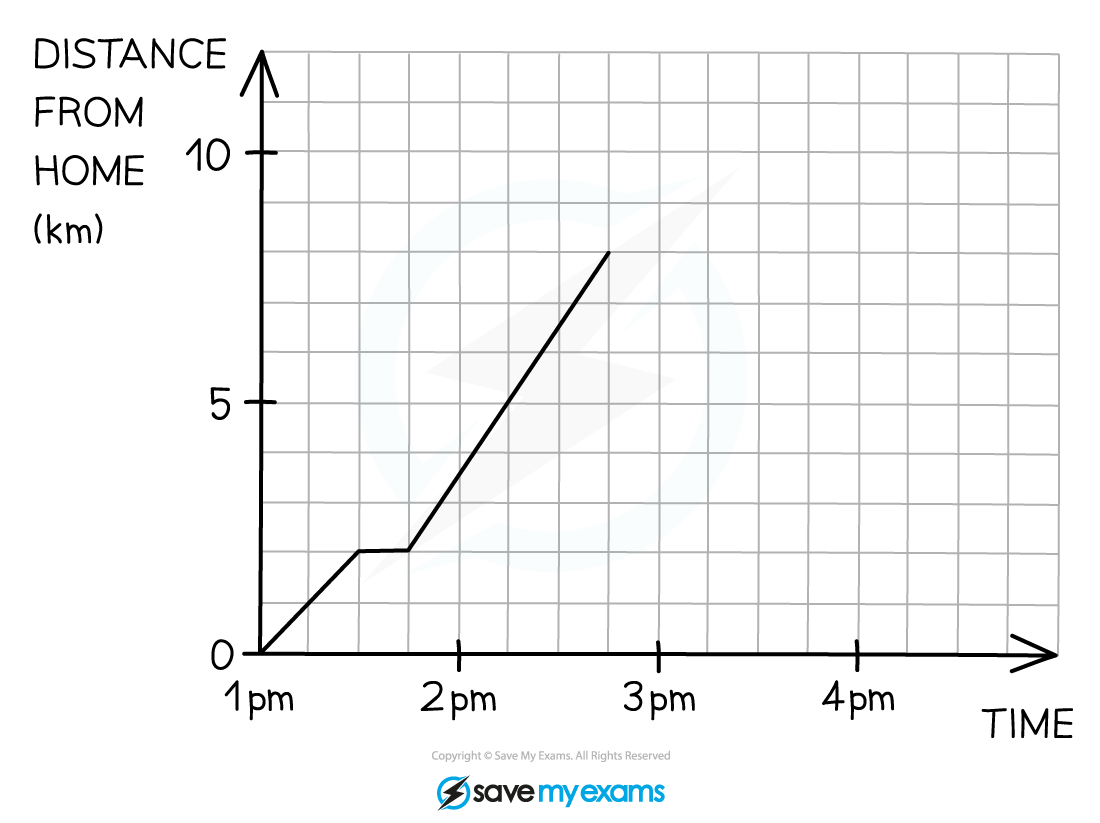#### Worked Example